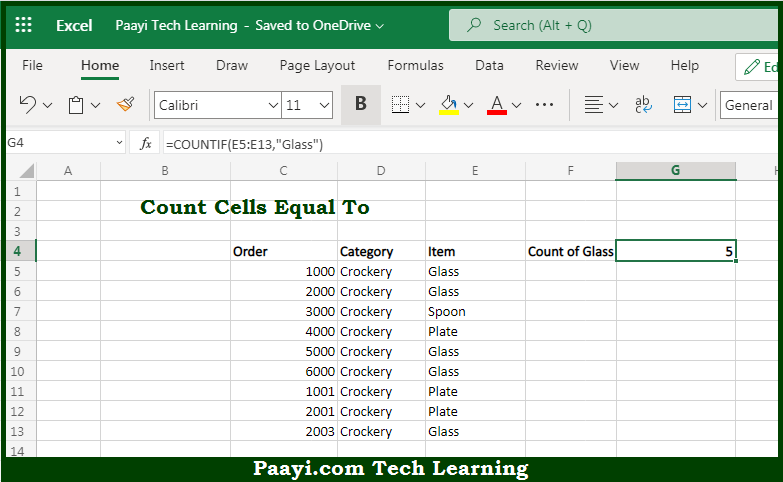# Learn How to Count Cells Equal To In Microsoft Excel

Written by | 0 Comments | 481 Views

In this article, you will learn how to use the Microsoft Excel COUNT formula and its purpose in Microsoft Excel. You will also get to know how to count cells equal to and see the generic formula.

Count Cells Equal To Using Microsoft Excel Count Formula

The main purpose of the Microsoft Excel COUNT formula is to count the number of cells equal to a specific value. Here we will learn how to count cells equal to a specific value.  That implies, with the help of the COUNT formula you can able to count the number of cells equal to a specific value, you can use the COUNTIF function. So, with the help of the COUNT formula, you can able to count the number of cells equal to a specific value.

General Syntax of the COUNT Formula To Count Cells Equal To

=COUNTIF(range,value)

The Explanation for the Count Cells Equal ToSo we know that Microsoft Excel COUNT formula you can able to count the number of cells equal to a specific value. Here we will learn how to count cells equal to a specific value. The COUNTIF function is has got the fully automatic features, and it counts the number of cells in a range that matches the provided or supplied criteria.

It should be noted that when the text values are supplied directly as criteria, is must be enclosed double quotes as (""). In case you are having criteria in another cell, you can supply the cell address as criteria without quotes. So, with the help of the COUNT formula, you can able to count the number of cells equal to a specific value.